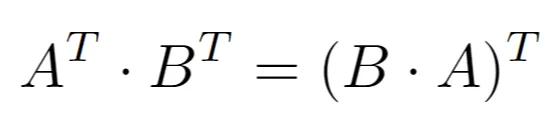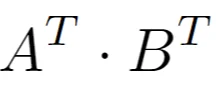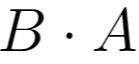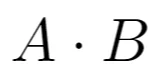15 Jun 2022

Posted on:

19 May 2022

0

# Last Example in Dot product for matrices

In the last example couldnt we transpose the 1st matrix to be 4x3 and transpose the 2nd to be 3x2 then we could get a 4x2 output matrix ?

Instructor
Posted on:

15 Jun 2022

0

Hey Ahmed,

Note the following equality:Therefore, transposing the matrices and then multiplying them would not give you the same result as multiplying them in the same order. The dot productsandwould yield a 4x2 and a 2x4 matrix, respectively. However, the matrices A and B, multiplied in the following orderare not compatible.

Hope this helps!

Kind regards,
365 Hristina